# LabVIEW Formula Nodes

The Formula Node in the LabVIEW software is a convenient, text-based node you can use to perform complicated mathematical operations on a block diagram using the C++ syntax structure. It is most useful for equations that have many variables or are otherwise complicated. The text-based code simplifies the block diagram and increases its readability. Furthermore, you can copy and paste existing code directly into the Formula Node rather than recreating it graphically.In addition to text-based equation expressions, the Formula Node can accept text-based versions of if statements, while loops, for loops, and do loops, which are familiar to C programmers. These programming elements are similar but not identical to those you find in C programming.

Using the Formula node

Complete the following steps to create a VI that computes different formulas depending on whether the product of the inputs is positive or negative.

1. Selecting File»New VI to open a blank VI.
2. Place a Formula Node on the block diagram.
1. Right-click on the diagram and navigate to Programming»Structures»Formula Node.
2. Click and drag the cursor to place the Formula Node on the block diagram.
3. Right-click the border of the Formula Node and select Add Input from the shortcut menu.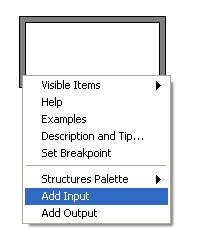1. Label the input variable x.
2. Repeat steps 3 and 4 to add another input and label it y.
3. Right-click the border of the Formula Node and select Add Output from the shortcut menu.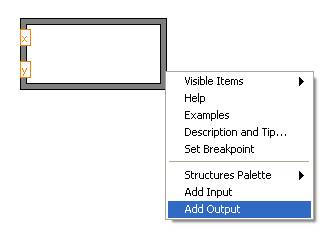1. Create two outputs and name them z1 and z2, respectively.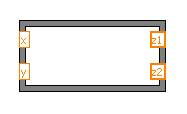1. Enter the expressions below in the Formula Node. Make sure that you complete each command with a semicolon. Notice, however, that the if statement does not require a semicolon after the first line.

if (x*y>0)
z1 = 3*x**2 – 2*y**3;
else z1 = 0;
z2 = sinh(z1);

1. Create controls and indicators for the inputs and outputs.
1. Right-click on each input and select Create»Control from the shortcut menu.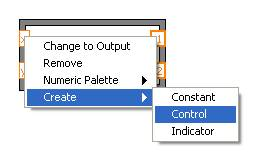2. Right-click on each output and select Create»Indicator from the shortcut menu.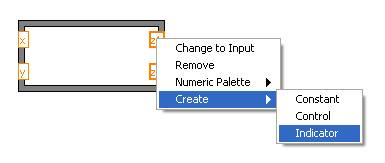1. Place a While Loop with a stop button around the Formula Node and the controls. Be sure to include a Wait (ms) function inside the loop to conserve memory usage. Your block diagram should appear as follows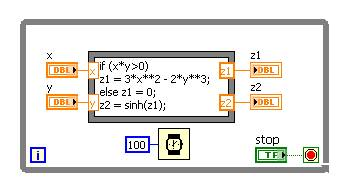1. Click the Run button to run the VI. Change the values of the input controls to see how the outputs change.

In this case, the Formula Node helps minimize the space required on the block diagram. Accomplishing the same task without the use of a Formula Node requires the following code.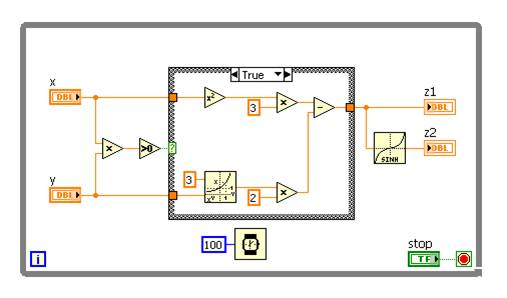Thank you for reading my blog post, and for more information on formula nodes follow this link. If you are interested in trying out LabVIEW for yourself, you can purchase a copy of LabVIEW 2014 Home Edition which includes everything you will need to run LINX 3.0. Please comment below with any questions or comments you may have.#### About Austin Stanton

View all posts by Austin Stanton →